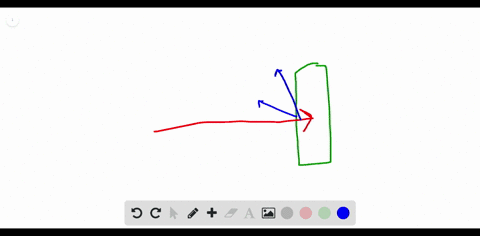### Discuss What experiment led to the dispute of J. …

00:20University of Miami
Problem 108

Formulate How were scientific methods used to determine the model of the atom? Why is the model considered a theory?

Scientific methods are used as:
- Observation.
- Forming a hypothesis.
- Testing the hypothesis.
- Formulating the theory.
- The model is considered a theory as it was tested by many scientists through years and their results proved the success of the theory.

## Discussion

You must be signed in to discuss.

## Video Transcript

So now we'll work on problem. Wanna wait from chapter for in this problem? Were asked, how were scientific methods used to determine the model of the atom? And why is this model considered theory? So scientific methods were used, um, in experiments such as, uh, the gold foil experiment or the cathode ray tube experiment. So researchers would create a publicist about what they expect to be the case, and they would test this hypothesis through the use of experiments like these and so based on their results. So based on the data that they obtained from thes experiments, they would evaluate their prophecies. Based on this experimental data, they would evaluate the about sees and propose models consistent with the results that they obtained. So the second part of this question is why is the model of an atom theory and the answer here is that it? Because it has not been, uh, confirmed. We haven't been able to directly observe the atomic structure oven Adam, since it is on such a small scale, and also because it has not been directly observed, we may obtain the future, uh, results from experiments which would suggest modifications to our models, so it hasn't been completely confirmed. It's not known with certainty, so it remains a theory. Until future data, um either modifies it work.